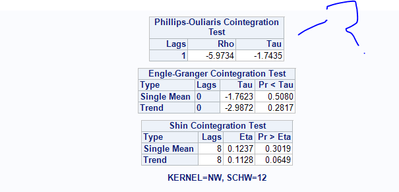## Phillips-Ouliaris Cointegration Test Results

Hello,

Following sample code gives me results and  I can interpret the Engle Granger results (attached). It implies the two series are not cointegrated. But how do I interpret the Phillips-Ouliaris test? Are the two series cointeggrate or not as per results the Phillipss-Ouliaris results?

proc autoreg data=dataset;
model Y = x1 / stationarity = (adf) stationarity = (PHILIPS) stationarity = (kpss);
run;
title1;1 ACCEPTED SOLUTION

Accepted Solutions

## Re: Phillips-Ouliaris Cointegration Test Results

Hello,

The AUTOREG procedure only produces the Phillips-Outliaris cointegration test statistics, rho and tau, it does not provide critical values or p values for the test.  You need to refer to the Phillips Outliaris (1990) paper tables Ia–Ic for the z_alpha  test and tables IIa–IIc for the z_t  test. The following section of the PROC AUTOREG documentation contains some discussions about the test which will be helpful to you to better understand the test and interpretation:

https://go.documentation.sas.com/doc/en/pgmsascdc/v_014/etsug/etsug_autoreg_syntax05.htm#etsug_autor...

then scroll down to:

STATIONARITY=(PHILLIPS)
STATIONARITY=(PHILLIPS=(value …value)

...

The marginal probabilities for cointegration testing are not produced. You can refer to Phillips and Ouliaris (1990) tables Ia–Ic for the Z_hat(alpha)  test and tables IIa–IIc for the Z_hat(t) test. The standard residual-based cointegration test can be obtained using the NOINT option in the MODEL statement, and the de-meaned test is computed by including the intercept term. To obtain the de-meaned and detrended cointegration tests, you should include the time trend variable in the regressors. For information about the Phillips-Ouliaris cointegration test, see Phillips and Ouliaris (1990) or Hamilton (1994, Tbl. 19.1). Note that Hamilton (1994, Tbl. 19.1) uses Z_rho  and Z_t instead of the original Phillips and Ouliaris (1990) notation. This chapter adopts the notation introduced in Hamilton. To distinguish from Student’s t distribution, these two statistics are named accordingly as  (rho) and  (tau).

In addition to the above section of the documentation, the following information will also be helpful to you to better understand how to use the tables provided in the paper:

On page 189 of the Philips and Ouliaris (1990) paper, the short paragraph with the title 'Usage', immediately above Table Ia, describes how to use the critical values in the table. The table consists of three subtables, namely, 'Standard', 'Demeaned', and 'Demeaned and Detrended', for both Z_rho (Table Ia, Ib, Ic) and Z_tau(Table IIa, IIb, Iic). Hamilton's Time Series Analysis book, page 599 contains detailed instruction on the three cases, which correspond to the three tables of critical values:

(1) If the estimated cointegrating regression does not contain any intercept, and none of the explanatory variables has a nonzero trend component, then use
Table Ia for Z_rho, and Table IIa for Z_tau ;

(2) If the estimated cointegrating regression contains an intercept, and none of the explantory variables has a nonzero trend component, then use Table Ib for Z_rho, and Table IIb for Z_tau ;

(3) If the estimated cointegrating regression contains an intercept, and at least one of the explanatory variables has a nonzero trend component, then use
Table Ic for Z_rho, and Table IIc for Z_tau.

For (3), the section 'Testing for Cointegration Among Trending Series' on page 596 of Hamilton's book will also be helpful to you.

Hope this helps.

## Re: Phillips-Ouliaris Cointegration Test Results

Hello,

The AUTOREG procedure only produces the Phillips-Outliaris cointegration test statistics, rho and tau, it does not provide critical values or p values for the test.  You need to refer to the Phillips Outliaris (1990) paper tables Ia–Ic for the z_alpha  test and tables IIa–IIc for the z_t  test. The following section of the PROC AUTOREG documentation contains some discussions about the test which will be helpful to you to better understand the test and interpretation:

https://go.documentation.sas.com/doc/en/pgmsascdc/v_014/etsug/etsug_autoreg_syntax05.htm#etsug_autor...

then scroll down to:

STATIONARITY=(PHILLIPS)
STATIONARITY=(PHILLIPS=(value …value)

...

The marginal probabilities for cointegration testing are not produced. You can refer to Phillips and Ouliaris (1990) tables Ia–Ic for the Z_hat(alpha)  test and tables IIa–IIc for the Z_hat(t) test. The standard residual-based cointegration test can be obtained using the NOINT option in the MODEL statement, and the de-meaned test is computed by including the intercept term. To obtain the de-meaned and detrended cointegration tests, you should include the time trend variable in the regressors. For information about the Phillips-Ouliaris cointegration test, see Phillips and Ouliaris (1990) or Hamilton (1994, Tbl. 19.1). Note that Hamilton (1994, Tbl. 19.1) uses Z_rho  and Z_t instead of the original Phillips and Ouliaris (1990) notation. This chapter adopts the notation introduced in Hamilton. To distinguish from Student’s t distribution, these two statistics are named accordingly as  (rho) and  (tau).

In addition to the above section of the documentation, the following information will also be helpful to you to better understand how to use the tables provided in the paper:

On page 189 of the Philips and Ouliaris (1990) paper, the short paragraph with the title 'Usage', immediately above Table Ia, describes how to use the critical values in the table. The table consists of three subtables, namely, 'Standard', 'Demeaned', and 'Demeaned and Detrended', for both Z_rho (Table Ia, Ib, Ic) and Z_tau(Table IIa, IIb, Iic). Hamilton's Time Series Analysis book, page 599 contains detailed instruction on the three cases, which correspond to the three tables of critical values:

(1) If the estimated cointegrating regression does not contain any intercept, and none of the explanatory variables has a nonzero trend component, then use
Table Ia for Z_rho, and Table IIa for Z_tau ;

(2) If the estimated cointegrating regression contains an intercept, and none of the explantory variables has a nonzero trend component, then use Table Ib for Z_rho, and Table IIb for Z_tau ;

(3) If the estimated cointegrating regression contains an intercept, and at least one of the explanatory variables has a nonzero trend component, then use
Table Ic for Z_rho, and Table IIc for Z_tau.

For (3), the section 'Testing for Cointegration Among Trending Series' on page 596 of Hamilton's book will also be helpful to you.

Hope this helps.

Discussion stats